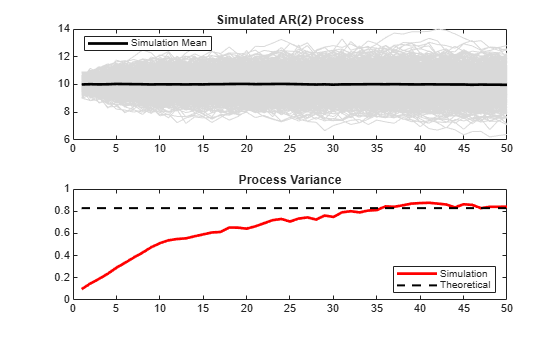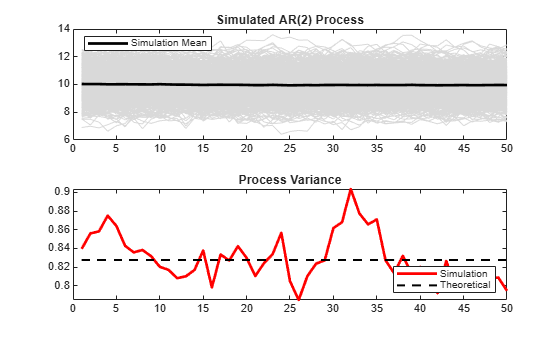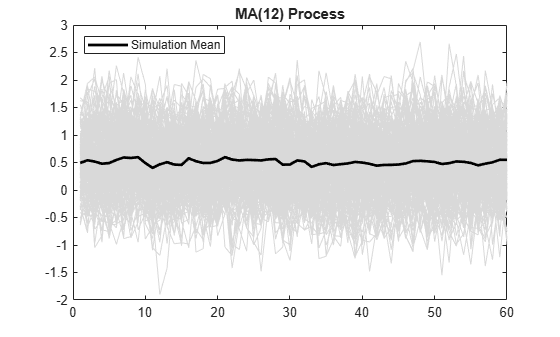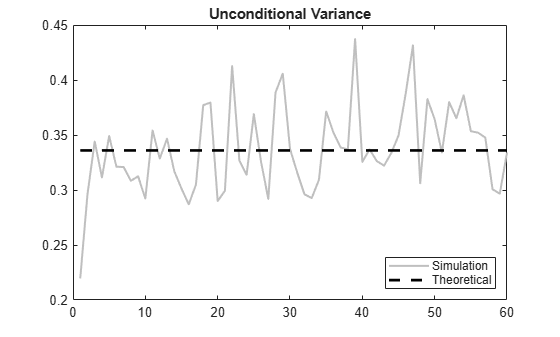## Simulate Stationary Processes

### Simulate AR Process

This example shows how to simulate sample paths from a stationary AR(2) process without specifying presample observations.

Create Model

Specify the AR(2) model

`${y}_{t}=0.5+0.7{y}_{t-1}+0.25{y}_{t-2}+{\epsilon }_{t},$`

where the innovation process is Gaussian with variance 0.1.

`Mdl = arima('Constant',0.5,'AR',{0.7 0.25},'Variance',.1);`

Generate One Sample Path

Generate one sample path (with 50 observations) from the specified model, and plot.

```rng(5) Y = simulate(Mdl,50); figure plot(Y) xlim([0,50]) title('Simulated AR(2) Process')```Because presample data was not specified, `simulate` sets the two required presample observations equal to the unconditional mean of the process

`$\mu =\frac{c}{\left(1-{\varphi }_{1}-{\varphi }_{2}\right)}.$`

The unconditional variance of the process is

`${\sigma }^{2}=\frac{\left(1-{\varphi }_{2}\right)}{\left(1+{\varphi }_{2}\right)}\frac{{\sigma }_{\epsilon }^{2}}{{\left(1-{\varphi }_{2}\right)}^{2}-{\varphi }_{1}^{2}}.$`

Compute the unconditional mean and variance of the process.

```c = Mdl.Constant; phi = Mdl.AR; sigmaEps2 = Mdl.Variance; mu = c/(1-phi{1}-phi{2})```
```mu = 10.0000 ```
`sigma2 = (1-phi{2})/(1+phi{2})*sigmaEps2/((1-phi{2})^2 - phi{1}^2)`
```sigma2 = 0.8276 ```

Generate Many Sample Paths

Generate 1000 sample paths, each with 50 observations.

```Y = simulate(Mdl,50,'NumPaths',1000); figure subplot(2,1,1) plot(Y,'Color',[.85,.85,.85]) title('Simulated AR(2) Process') hold on h = plot(mean(Y,2),'k','LineWidth',2); legend(h,'Simulation Mean','Location','NorthWest') hold off subplot(2,1,2) plot(var(Y,0,2),'r','LineWidth',2) title('Process Variance') hold on plot(1:50,sigma2*ones(50,1),'k--','LineWidth',1.5) legend('Simulation','Theoretical',... 'Location','SouthEast') hold off```The simulation mean is constant over time. This is consistent with the definition of a stationary process. The process variance is not constant over time, however. There are transient effects at the beginning of the simulation due to the absence of presample data.

Around observation 50, the simulated variance approaches the theoretical variance.

Oversample to Reduce Transient Effects

To reduce transient effects, one option is to oversample the process. For example, to sample 50 observations, you can generate paths with more than 50 observations, and discard all but the last 50 observations as burn-in. Here, simulate paths of length 150, and discard the first 100 observations.

```Y = simulate(Mdl,150,'NumPaths',1000); Y = Y(101:end,:); figure subplot(2,1,1) plot(Y,'Color',[.85,.85,.85]) title('Simulated AR(2) Process') hold on h = plot(mean(Y,2),'k','LineWidth',2); legend(h,'Simulation Mean','Location','NorthWest') hold off subplot(2,1,2) plot(var(Y,0,2),'r','LineWidth',2) xlim([0,50]) title('Process Variance') hold on plot(1:50,sigma2*ones(50,1),'k--','LineWidth',1.5) legend('Simulation','Theoretical',... 'Location','SouthEast') hold off```The realizations now look like draws from a stationary stochastic process. The simulation variance fluctuates (due to Monte Carlo error) around the theoretical variance.

### Simulate MA Process

This example shows how to simulate sample paths from a stationary MA(12) process without specifying presample observations.

Create Model

Specify the MA(12) model

`${y}_{t}=0.5+{\epsilon }_{t}+0.8{\epsilon }_{t-1}+0.2{\epsilon }_{t-12},$`

where the innovation distribution is Gaussian with variance 0.2.

```Mdl = arima('Constant',0.5,'MA',{0.8,0.2},... 'MALags',[1,12],'Variance',0.2);```

Generate Sample Paths

Generate 200 sample paths, each with 60 observations.

```rng(5) Y = simulate(Mdl,60,'NumPaths',200); figure plot(Y,'Color',[.85,.85,.85]) hold on h = plot(mean(Y,2),'k','LineWidth',2); legend(h,'Simulation Mean','Location','NorthWest') title('MA(12) Process') hold off```For an MA process, the constant term is the unconditional mean. The simulation mean is approximately $\mathit{c}$ = 0.5.

Plot Simulation Variance

The unconditional variance of the process is

`${\sigma }^{2}=\left(1+{\theta }_{1}^{2}+{\theta }_{12}^{2}\right){\sigma }_{\epsilon }^{2}.$`

Compute the unconditional variance.

```theta = cell2mat(Mdl.MA); sigmaEps2 = Mdl.Variance; sigma2 = (1+sum(theta.^2))*sigmaEps2```
```sigma2 = 0.3360 ```

Because the model is stationary, the unconditional variance should be constant across all times. Plot the simulation variance, and compare it to the theoretical variance.

```figure plot(var(Y,0,2),'Color',[.75,.75,.75],'LineWidth',1.5) xlim([0,60]) title('Unconditional Variance') hold on plot(1:60,sigma2*ones(60,1),'k--','LineWidth',2) legend('Simulation','Theoretical',... 'Location','SouthEast') hold off```There appears to be a short burn-in period at the beginning of the simulation. During this time, the simulation variance is lower than expected. Afterwards, the simulation variance fluctuates around the theoretical variance.

Generate Many Sample Paths

Simulate 10,000 paths from the model, each with length 1000. Plot the simulation variance.

```YM = simulate(Mdl,1000,'NumPaths',10000); figure plot(var(YM,0,2),'Color',[.75,.75,.75],'LineWidth',1.5) ylim([0.3,0.36]) title('Unconditional Variance') hold on plot(1:1000,sigma2*ones(1000,1),'k--','LineWidth',2) legend('Simulation','Theoretical',... 'Location','SouthEast') hold off```The Monte Carlo error is reduced when more realizations are generated. There is much less variability in the simulation variance, which tightly fluctuates around the theoretical variance.